# Chapter 2 Motion of Charged Particles in Fields

Plasmas are complicated because motions of electrons and ions are determined by the electric and magnetic fields but also change the fields by the currents they carry.
For now we shall ignore the second part of the problem and assume that Fields are Prescribed.
Even so, calculating the motion of a charged particle can be quite hard.
Equation of motion:

 m dv dt

Rate of change of momentum

=

 q charge ( E E−field + v velocity ∧ B B−field )

Lorentz Force

(2.1)
Have to solve this differential equation, to get position r and velocity (v= · r) given E(r,t), B(r,t).
Approach here: Start simple, gradually generalize. For a more sophisticated demonstration that the simple results are comprehensive see http://silas.psfc.mit.edu/introplasma/drifts.pdf.

## 2.1  Uniform B field, E = 0.

 m ⋅ v = q v∧B
(2.2)

### 2.1.1  Qualitatively

in the plane perpendicular to B: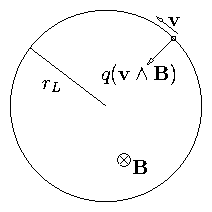Figure 2.1: Circular orbit in uniform magnetic field.
Accel. is perp to v so particle moves in a circle whose radius rL is such as to satisfy
 m rL Ω2 = m v⊥2 rL = |q |v⊥ B
(2.3)
Ω is the angular (velocity) frequency
1st equality shows Ω2 = v2 /rL2   (rL = v / Ω)
Hence second gives m v Ω = |q |v B
 i.e.    Ω  = |q |B m .
(2.4)
Particle moves in a circular orbit with

 angular frequency   Ω = |q |B m the "Cyclotron Frequency"
(2.5)

(2.6)

### 2.1.2  By Vector Algebra

• Particle Energy is constant. proof: take v. Eq. of motion then
 m v. ⋅ v = d dt ⎛⎝ 1 2 m v2 ⎞⎠ = q v. (v∧B) = 0 .
(2.7)
• Parallel and Perpendicular motions separate. v|| = constant because accel ( ∝ vB) is perpendicular to B.
Perpendicular Dynamics:
Take B in z direction and write components
 m ⋅ v x = q vy B   ,   m ⋅ v y = − q vx B
(2.8)
Hence
 ⋅⋅ v x = qB m ⋅ v y = − ⎛⎝ qB m ⎞⎠ 2 vx = − Ω2 vx
(2.9)
Solution: vx = v cosΩt    (choose zero of time)
Substitute back:
 vy = m qB ⋅ v x = − |q | q v⊥ sinΩt
(2.10)
Integrate:
 x = x0 + v⊥ Ω sinΩt    ,    y   =  y0 + q |q | v⊥ Ω cosΩt
(2.11)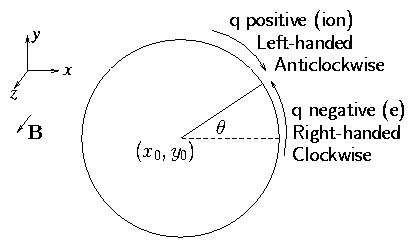Figure 2.2: Gyro center (x0,y0) and orbit projection viewed from positive z.
This is the equation of a circle with center r0 = (x0, y0) and radius rL = v/ Ω: Gyro Radius. [Angle is θ = Ωt]
Direction of rotation is as indicated in Fig. 2.2 for opposite sign of charge:
Ions rotate anticlockwise. Electrons clockwise about the magnetic field.
The current carried by the plasma always is in such a direction as to reduce the magnetic field.
This is the property of a magnetic material which is "Diamagnetic".
When v|| is non-zero the total motion is along a helix.

## 2.2  Uniform B and non-zero E

 m ⋅ v = q (E+ v∧B)
(2.12)
Parallel motion: Before, when E = 0 this was v|| = const. Now it is clearly
 ⋅ v || = q E|| m
(2.13)
Constant acceleration along the field.
Perpendicular Motion
Qualitatively: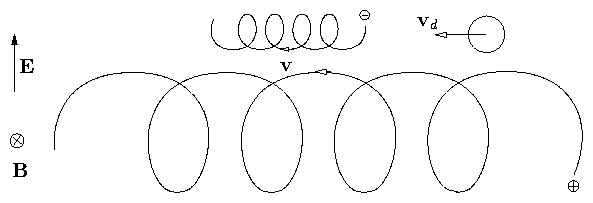Figure 2.3: EB drift orbit
Speed of positive particle is greater at top than bottom so radius of curvature is greater. See Fig. 2.3. Result is that guiding center moves perpendicular to both E and B. It `drifts' across the field.
Algebraically:   It is clear that if we can find a constant velocity vd that satisfies
 E+ vd ∧B= 0
(2.14)
then the sum of this drift velocity plus the velocity
 vL = d dt [rL eiΩ(t−t0)]
(2.15)
which we calculated for the E = 0 gyration will satisfy the equation of motion.
Take ∧B the above equation:
 0 = E∧B+ (vd ∧B) ∧B=E∧B+ (vd . B) B− B2 vd
(2.16)
so that
 vd = E∧B B2
(2.17)
does satisfy it.
Hence the full solution is
 v= v|| parallel + vd                     cross−field drift + vL                     Gyration
(2.18)
where
 ⋅ v || = q E|| m
(2.19)
and
vd (eq 2.17) is the "E × B drift" of the gyrocenter.
Comments on E × B drift:
1. It is independent of the properties of the drifting particle (q, m, v, whatever).
2. Hence it is in the same direction for electrons and ions.
3. Underlying physics for this is that in the frame moving at the E × B drift E = 0. We have `transformed away' the electric field.
4. Formula given above is exact except for the fact that relativistic effects have been ignored. They would be important if vd  ∼ c.

### 2.2.1  Drift due to Gravity or other Forces

Suppose particle is subject to some other force, such as gravity. Write it F so that
 m ⋅ v = F + q v∧B = q ( 1 q F+ v∧B)
(2.20)
This is just like the Electric field case except with F/q replacing E.
The drift is therefore
 vd = 1 q F ∧B B2
(2.21)
In this case, if force on electrons and ions is same, they drift in opposite directions.
This general formula can be used to get the drift velocity in some other cases of interest (see later).

## 2.3  Non-Uniform B Field

If B-lines are straight but the magnitude of B varies in space we get orbits that look qualitatively similar to the E ⊥ B case: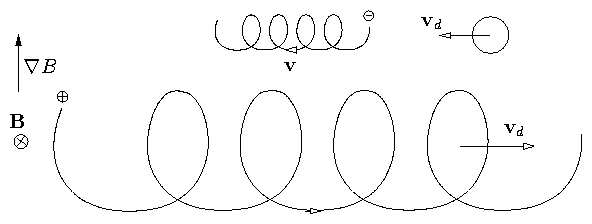Figure 2.4: ∇B drift orbit
Curvature of orbit is greater where B is greater causing loop to be small on that side. Result is a drift perpendicular to both B and ∇B. Notice, though, that electrons and ions go in opposite directions (unlike EB).

#### Algebra

We try to find a decomposition of the velocity as before into v = vd + vL where vd is constant.
We shall find that this can be done only approximately. Also we must have a simple expression for B. This we get by assuming that the Larmor radius is much smaller than the scale length of B variation i.e.,
 rL << B/ |∇ B |
(2.22)
in which case we can express the field approximately as the first two terms in a Taylor expansion:
 B ≅ B0 + (r. ∇ ) B
(2.23)
Then substituting the decomposed velocity we get:
 m dv dt
 =
 m ⋅ v L = q (v∧B) = q [ vL ∧B0 + vd ∧B0 + (vL + vd) ∧(r. ∇) B]
(2.24)
 or    0
 =
 vd ∧B0 + vL ∧(r. ∇ ) B+ vd ∧(r. ∇) B
(2.25)
Now we shall find that vd/vL is also small, like r| ∇B | /B. Therefore the last term here is second order but the first two are first order. So we drop the last term.
Now the awkward part is that vL and rL are periodic. Substitute r = rc + rL where rc is the center of the orbit (gyrocenter), the nonperiodic part; then
 0 = vd ∧B0 + vL ∧(rL . ∇ ) B+ vL ∧( rc . ∇ ) B
(2.26)
We now average over a cyclotron period. The last term is ∝ e−iΩt so it averages to zero:
 0 = vd ∧B+ 〈vL ∧(rL . ∇ ) B〉 .
(2.27)
To perform the average use

 rL = ( xL , yL)
 =
 v⊥ Ω ⎛⎝ sinΩt , q |q | cosΩt ⎞⎠
(2.28)
 vL = ( ⋅ x L , ⋅ y L )
 =
 v⊥ ⎛⎝ cosΩt , −q |q | sinΩt ⎞⎠
(2.29)
 So     [ vL ∧(r. ∇ ) B]x
 =
 vy y d dy B
(2.30)
 [ vL ∧( r. ∇ ) B]y
 =
 −vx y d dy B,
(2.31)
taking ∇B to be in the y-direction.
Then
 〈vy y 〉
 =
 − 〈cosΩt sinΩt 〉 v⊥2 Ω = 0
(2.32)
 〈vx y 〉
 =
 q |q | 〈cosΩt cosΩt 〉 v⊥2 Ω = 1 2 v⊥2 Ω q |q |
(2.33)
So
 〈vL ∧(r. ∇ ) B〉 = − q |q | 1 2 v⊥2 Ω ∇ B
(2.34)
Substitute in:
 0 = vd ∧B− q |q | v⊥2 2Ω ∇ B
(2.35)
and solve as before to get
vd =
 ⎛⎝ −q |q | v⊥2 2 Ω ∇ B ⎞⎠ ∧B

B2
= q

|q |
v2

2 Ω
B∧∇ B

B2
(2.36)
or equivalently
 vd = 1 q mv⊥2 2 B B∧∇ B B2
(2.37)
This is called the `Grad B drift'.

## 2.4  Curvature Drift

When the B-field lines are curved and the particle has a velocity v|| along the field, another drift occurs.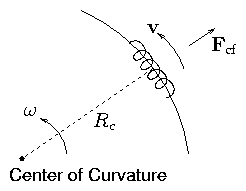Figure 2.5: Curvature and Centrifugal Force
Take |B| constant; radius of curvature Rc.
To 1st order the particle just spirals along the field.
In the frame of the guiding center a force appears because the plasma is rotating about the center of curvature.
This centrifugal force is Fcf
 Fcf = m v||2 Rc pointing outward
(2.38)
as a vector
 Fcf = m v||2 Rc Rc2
(2.39)
[There is also a coriolis force 2m(ω ∧v) but this averages to zero over a gyroperiod.]
Use the previous formula for a force
 vd = 1 q Fcf ∧B B2 = m v||2 q B2 Rc ∧B Rc2
(2.40)
This is the "Curvature Drift".
It is often convenient to have this expressed in terms of the field gradients. So we relate Rc to ∇B etc. as follows: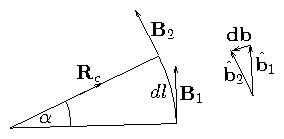Figure 2.6: Differential expression of curvature
(Carets denote unit vectors)
From the diagram
 db = ^ b 2 − ^ b 1 = − ^ R c α
(2.41)
and
 d l =  αRc
(2.42)
So
d b

d l
= −
 ^ R c

Rc
= − Rc

Rc2
(2.43)
But (by definition)
 d b d l = ( ^ b . ∇ ) ^ b
(2.44)
So the curvature drift can be written
vd = m v||2

q
Rc

Rc2
B

B2
= mv||2

q
 B∧( ^ b . ∇ ) ^ b

B2
(2.45)

### 2.4.1  Vacuum Fields

Relation between ∇ B & Rc drifts
The curvature and ∇B are related because of Maxwell's equations, their relation depends on the current density j. A particular case of interest is j=0: vacuum fields.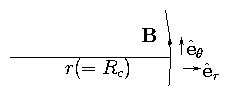Figure 2.7: Local polar coordinates in a vacuum field

 ∇ ∧B= 0     (static case)
(2.46)
Consider the z-component
 0 = ( ∇ ∧B)z
 =
 1 r ∂ ∂r (r Bθ )   (Br = 0  by choice).
(2.47)
 = ∂Bθ ∂r
 +
 Bθ r
(2.48)
or, in other words,
 ( ∇ B )r = − B Rc
(2.49)
[Note also 0 = (∇ ∧B)θ = ∂Bθ/∂z : (∇ B )z = 0]
and hence (∇ B)perp = − B Rc/Rc2.
Thus the grad B drift can be written:
 v∇B = mv⊥2 2 q B∧∇ B B3 = mv⊥2 2 q Rc ∧B Rc2 B2
(2.50)
and the total drift across a vacuum field becomes
 vR + v∇B = 1 q ⎛⎝ mv||2 + 1 2 mv⊥2 ⎞⎠ Rc ∧B Rc2 B2 .
(2.51)
Notice the following:
1. Rc & ∇B drifts are in the same direction.
2. They are in opposite directions for opposite charges.
3. They are proportional to particle energies
4. Curvature ↔ Parallel Energy (× 2)
∇B ↔ Perpendicular Energy
5. As a result one can very quickly calculate the average drift over a thermal distribution of particles because
 〈 1 2 m v||2 〉
 =
 T 2
(2.52)
 〈 1 2 m v⊥2 〉
 =
 T       2 degrees of freedom
(2.53)
Therefore
vR + v∇B 〉 = 2T

q
RcB

Rc2 B2

= 2T

q
 B∧ ⎛⎝ ^ b . ∇ ⎞⎠ ^ b

B2

(2.54)

## 2.5  Interlude: Toroidal Confinement of Single Particles

Since particles can move freely along a magnetic field even if not across it, we cannot obviously confine the particles in a straight magnetic field. Obvious idea: bend the field lines into circles so that they have no ends.Figure 2.8: Toroidal field geometry
Problem
Curvature & ∇B drifts

 vd
 =
 1 q ⎛⎝ mv||2 + 1 2 mv⊥2 ⎞⎠ R ∧B R2 B2
(2.55)
 |vd |
 =
 1 q ⎛⎝ mv||2 + 1 2 mv⊥2 ⎞⎠ 1 BR
(2.56)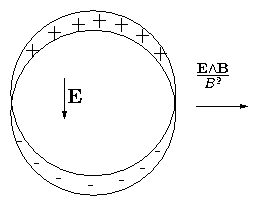Figure 2.9: Charge separation due to vertical drift
Ions drift up. Electrons down. There is no confinement. When there is finite density things are even worse because charge separation occurs → E → E ∧B → Outward Motion.

### 2.5.1  How to solve this problem?

Consider a beam of electrons v|| ≠ 0  v = 0. Drift is
 vd = mv||2 q 1 BTR
(2.57)
What Bz is required to cancel this?
Adding Bz gives a compensating vertical velocity
 v = v|| Bz BT for Bz   <<  BT
(2.58)
We want total
 vz = 0 = v|| Bz BT + mv||2 q 1 BTR
(2.59)
So Bz = − mv||/ Rq is the right amount of field.
Note that this is such as to make
 rL (Bz) = |mv|| | |q Bz | = R   .
(2.60)
But Bz required depends on v|| and q so we can't compensate for all particles simultaneously.
Vertical field alone cannot do it.

### 2.5.2  The Solution: Rotational Transform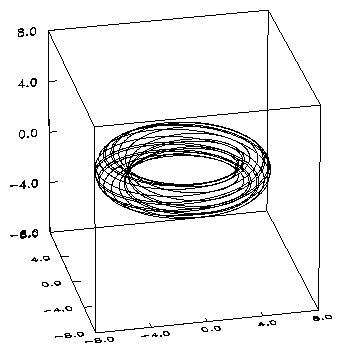Figure 2.10: Tokamak field lines with rotational transform
Toroidal Coordinate system (r, θ, ϕ) (minor radius, poloidal angle, toroidal angle), see figure 2.8.
Suppose we have a poloidal field Bθ
Field Lines become helical and wind around the torus: figure 2.10.
In the poloidal cross-section the field describes a circle as it goes round in ϕ.
Equation of motion of a particle exactly following the field is:
 r d θ dt = Bθ Bϕ vϕ = Bθ Bϕ Bϕ B v||  = Bθ B v||
(2.61)
and
 r = constant.
(2.62)
Now add on to this motion the cross field drift in the z direction.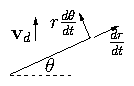Figure 2.11: Components of velocity

 r d θ dt
 =
 Bθ B v|| + vd cosθ
(2.63)
 dr dt
 =
 vd sinθ
(2.64)
Take ratio, to eliminate time:
1

r
dr

= vd sinθ

 Bθ B v|| +vd cosθ
(2.65)
Take Bθ, B, v||, vd to be constants, then we can integrate this orbit equation:
 [ lnr ] = [ − ln| Bθ v|| B + vd cosθ|]  .
(2.66)
Take r = r0 when cosθ = 0 (θ = [( π)/2]) then
 r = r0 / ⎡⎣ 1 + Bvd Bθ v|| cosθ ⎤⎦
(2.67)
If [( Bvd)/(Bθ v|| )] << 1 this is approximately
 r = r0 − ∆cosθ
(2.68)
where ∆ = [(Bvd)/(Bθ v|| )] r0
This is approximately a circular orbit shifted by a distance ∆: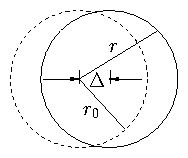Figure 2.12: Shifted, approximately circular orbit
Substitute for vd
 ∆
 ≅
r0 B

Bθ
1

q
 (mv||2 + 1 2 mv⊥2)

v||
1

Bϕ R
(2.69)
 ≅
1

q Bθ
 mv||2 + 1 2 mv⊥2

v||
r0

R
(2.70)
 If  v⊥ = 0
 ∆ = mv|| q Bθ r0 R = rLθ r0 R ,
(2.71)
where r is the Larmor Radius in a field Bθ ×r / R.
Provided ∆ is small, particles will be confined. Obviously the important thing is the poloidal rotation of the field lines: Rotational Transform.

#### Rotational Transform

 rotational transform
 ≡
 poloidal angle 1 toroidal  rotation
(2.72)
 ( transform / 2π = )
 ι ≡
 poloidal angle toroidal angle .
(2.73)
(Originally, ι was used to denote the transform. Since about 1990 it has been used to denote the transform divided by 2π which is the inverse of the safety factor.)

#### `Safety Factor'

 qs = 1 ι = toroidal angle poloidal angle .
(2.74)
Actually the value of these ratios may vary as one moves around the magnetic field. Definition strictly requires one should take the limit of a large no. of rotations.
qs is a topological number: number of rotations the long way per rotation the short way.
Cylindrical approx.:
 qs = r Bϕ R Bθ
(2.75)
In terms of safety factor the orbit shift can be written
 |∆| = rL θ r R = rL ϕ Bϕ r Bθ R = rL qs
(2.76)
(assuming Bϕ >> Bθ).

## 2.6  The Mirror Effect of Parallel Field Gradients: E = 0, ∇B ||B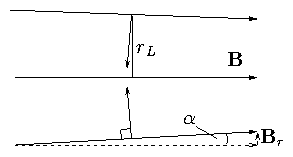Figure 2.13: Basis of parallel mirror force
In the above situation there is a net force along B.
Force is
 < F|| >
 =
 − |q v∧B| sinα =  − |q |v⊥ B sinα
(2.77)
 sinα
 =
 − Br B
(2.78)
Calculate Br as function of Bz from ∇ . B = 0.
 ∇ . B= 1 r ∂ ∂r (rBr) + ∂ ∂z Bz = 0  .
(2.79)
Hence
 [rBr] = − ⌠⌡ r ∂Bz ∂z dr
(2.80)
Suppose rL is small enough that [(∂Bz)/(∂z)] ≅ const.
 [ r Br]0rL ≅ − ⌠⌡ rL 0 r dr ∂Bz ∂z = − 1 2 rL2 ∂Bz ∂z
(2.81)
So
 Br(rL) = − 1 2 rL ∂Bz ∂z
(2.82)

 sinα = − Br B = + rL 2 1 B ∂Bz ∂z
(2.83)
Hence
< F|| > = − |q |  v rL

2
∂Bz

∂z
= −
 1 2 mv⊥2

B
∂Bz

∂z
.
(2.84)
As particle enters increasing field region it experiences a net parallel retarding force.
Define Magnetic Moment
 μ ≡ 1 2 m v⊥2 / B  .
(2.85)
Note this is consistent with loop current definition
 μ = A I = πrL2 . |q |v⊥ 2 πrL = |q |rL v⊥ 2
(2.86)
Force is F|| = μ . ∇|| B
This is force on a `magnetic dipole' of moment μ.
 F|| = μ . ∇|| B
(2.87)
Our μ always points along B but in opposite direction.

### 2.6.1  Force on an Elementary Magnetic Moment Circuit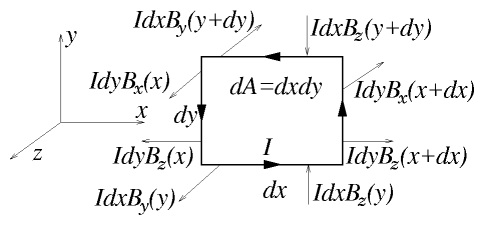Figure 2.14: Force components on an elementary circuit constituting a magnetic dipole.
Consider a plane rectangular circuit carrying current I having elementary area dxdy = dA. Regard this as a vector pointing in the z direction dA. The force on this circuit in a field B(r) is F such that
 Fx
 =
 Idy [Bz (x+dx) − Bz(x)] = I dydx ∂Bz ∂x
(2.88)
 Fy
 =
 −I dx [Bz (y+dy) − Bz(y)] = I dydx ∂Bz ∂y
(2.89)
 Fz
 =
 − I dx [By (y+dy) − By(y)] − I dy[Bx(x+dx) − Bx(x)]
(2.90)
 =
 − I dxdy ⎡⎣ ∂Bx ∂x + ∂By ∂y ⎤⎦ = I dydx ∂Bz ∂z
(2.91)
(Using ∇ . B = 0).
Hence, summarizing: F = I dydx ∇ Bz. Now define μ = I dA = I dydx z and take it constant. Then clearly the force can be written
 F = ∇ (B. μ )   [ Strictly = (∇ B) . μ]
(2.92)
μ is the (vector) magnetic moment of the circuit.
The shape of the circuit does not matter since any circuit can be considered to be composed of the sum of many rectangular circuits. So in general
 μ = I dA
(2.93)
and force is
 F = ∇ (B. μ)         (μ constant),
(2.94)
We shall show in a moment that |μ | is constant for a circulating particle, regarded as an elementary circuit. Also, μ for a particle always points in the -B direction. [Note that this means that the effect of particles on the field is to decrease it.] Hence the force may be written
 F = − μ∇ B
(2.95)
This gives us both:
• Magnetic Mirror Force:
 F|| = −μ∇||B
(2.96)
and
 v∇B = 1 q F ∧B B2 = μ q B∧∇ B B2 .
(2.97)

### 2.6.2  μ is a constant of the motion

#### Proof from F||

Parallel equation of motion
 m dv|| dt = F|| = − μ dB dz
(2.98)
So
 m v|| dv|| dt = d dt ( 1 2 m v||2 ) = − μvz dB dz = − μ dB dt
(2.99)
or
 d dt ( 1 2 m v||2) + μ dB dt = 0   .
(2.100)
Conservation of Total KE
 d dt ( 1 2 mv||2 + 1 2 mv⊥2 ) = 0
(2.101)
 =
 d dt ( 1 2 mv||2 + μB) = 0
(2.102)
Combine
 d dt (μB) − μ dB dt = 0
(2.103)

 = d μ dt = 0            As required
(2.104)

#### Angular Momentum

of particle about the guiding center is
 rL mv⊥
 =
mv

|q |B
m v = 2m

|q |
 1 2 mv⊥2

B
(2.105)
 =
 2m |q | μ   .
(2.106)
Conservation of magnetic moment is basically conservation of angular momentum about the guiding center.

#### Proof direct from Angular Momentum

Consider angular momentum about G.C. Because θ is ignorable (locally) Canonical angular momentum is conserved.
 p = [ r ∧( m v+ q A) ]z         conserved.
(2.107)
Here A is the vector potential such that B = ∇ ∧A
the definition of the vector potential means that
 Bz
 =
 1 r ∂(rAθ)) ∂r
(2.108)
 ⇒ rL Aθ (rL)
 =
 ⌠⌡ rL 0 r . Bz dr = rL2 2 Bz = μm |q |
(2.109)
Hence
 p
 =
 − q |q | rL v⊥ m + q mμ |q|
(2.110)
 =
 − q |q | m μ.
(2.111)
So p = const ↔ μ = constant.
Conservation of μ is basically conservation of angular momentum of particle about G.C.

### 2.6.3  Mirror Trapping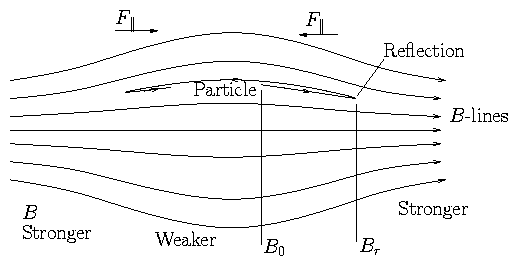Figure 2.15: Magnetic Mirror
F|| may be enough to reflect particles back. But may not!
Let's calculate whether it will:
Suppose reflection occurs.
At reflection point v||r = 0.
Energy conservation
 1 2 m (v⊥02 + v||02) = 1 2 m v⊥r2
(2.112)
μ conservation
 1 2 m v⊥02

B0
=
 1 2 m v⊥r2

Br
(2.113)
Hence
 v⊥02 + v||02
 =
 Br B0 v⊥02
(2.114)
 B0 Br
 =
 v⊥02 v⊥02 + v||o2
(2.115)

#### Pitch Angle    θ

 tanθ
 =
 v⊥ v||
(2.116)
 B0 Br
 =
 v⊥02 v⊥02 +v||02 = sin2 θ0
(2.117)
So, given a pitch angle θ0, reflection takes place where B0/Br = sin2θ0.
If θ0 is too small no reflection can occur.
Critical angle θc is obviously
 θc = sin−1 (B0 / B1)1/2
(2.118)
Loss Cone is all θ < θc.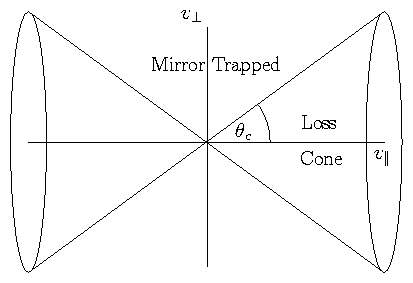Figure 2.16: Critical angle θc divides velocity space into a loss-cone and a region of mirror-trapping
Importance of Mirror Ratio: Rm = B1 / B0.

### 2.6.4  Other Features of Mirror Motions

Flux enclosed by gyro orbit is constant.
 Φ
 =
 πrL2 B = πm2 v⊥2 q2 B2 B
(2.119)
 =
2 πm

q2
 1 2 mv⊥2

B
(2.120)
 =
 2 πm q2 μ = constant.
(2.121)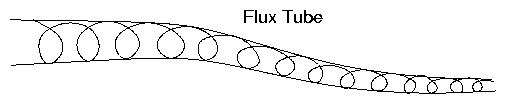Figure 2.17: Flux tube described by orbit
Note that if B changes `suddenly' μ might not be conserved.
Basic requirement
 rL << B / |∇B |
(2.122)
Slow variation of B (relative to rL).

## 2.7  Time Varying B Field     (E inductive)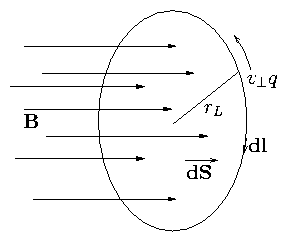Figure 2.18: Particle orbits round B so as to perform a line integral of the Electric field
Particle can gain energy from the inductive E field
 ∇∧E
 =
 − ∂B ∂t
(2.123)
 or ⌠(⎜)⌡ E. d l
 =
 − ⌠⌡ s ⋅ B . ds = − d Φ d t
(2.124)
Hence work done on particle in 1 revolution is
 δw = − ⌠(⎜)⌡ |q|E. dl = + |q | ⌠⌡ s ⋅ B . ds = + |q | d Φ dt = |q | ⋅ B πrL2
(2.125)
(dl and v q are in opposition directions).

 δ ⎛⎝ 1 2 m v⊥2 ⎞⎠
 =
|q |

B

πr L2 =
 2 π ⋅ B m

|q |B
 1 2 m v⊥2

B
(2.126)
 =
 2 π ⋅ B

|Ω|
μ.
(2.127)
Hence
 d dt ⎛⎝ 1 2 m v⊥2 ⎞⎠ = |Ω| 2 π δ ⎛⎝ 1 2 mv⊥2 ⎞⎠ = μ dB dt
(2.128)
but also
 d dt ⎛⎝ 1 2 mv⊥2 ⎞⎠ = d dt ( μB )  .
(2.129)
Hence
 dμ dt = 0 .
(2.130)
Notice that since Φ = [(2 πm)/(q2 )] μ, this is just another way of saying that the flux through the gyro orbit is conserved.
Notice also energy increase. Method of `heating'. Adiabatic Compression.

## 2.8  Time Varying E-field     (E, B uniform)

Recall the EB drift:
 vE ∧B = E∧B B2
(2.131)
when E varies so does vE ∧B. Thus the guiding centre experiences an acceleration
 ⋅ v E ∧B = d dt ⎛⎝ E∧B B2 ⎞⎠
(2.132)
In the frame of the guiding centre which is accelerating, a force is felt.
 Fa = − m d dt ⎛⎝ E∧B B2 ⎞⎠ (Pushed  back  into  seat!  −ve.)
(2.133)
This force produces another drift
 vD
 =
 1 q Fa ∧B B2 = − m qB2 d dt ⎛⎝ E∧B B2 ⎞⎠ ∧B
(2.134)
 =
 − m qB2 d dt ⎛⎝ ⎛⎝ E. ^ b ⎞⎠ ^ b −E ⎞⎠
(2.135)
 =
 m q B2 ⋅ E ⊥
(2.136)
This is called the `polarization drift'.

 vD = vE ∧B
 +
 vp = E∧B B2 + m q B2 ⋅ E ⊥
(2.137)
 =
 E ∧B B2 + 1 ΩB ⋅ E ⊥
(2.138)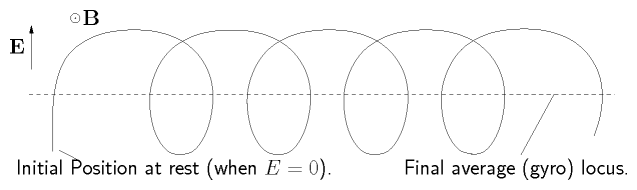Figure 2.19: Suddenly turning on an electric field causes a shift of the gyrocenter in the direction of force. This is the polarization drift.
Start-up effect: When we `switch on' an electric field the average position (gyro center) of an initially stationary particle shifts over by  ∼ 1/2 the orbit size. The polarization drift is this polarization effect on the medium.
Total shift due to vp is
 ∆r = ⌠⌡ vp dt = m q B2 ⌠⌡ ^ E ⊥ dt = m q B2 [ ∆E⊥ ]    .
(2.139)

### 2.8.1  Direct Derivation of [(dE)/dt] effect: `Polarization Drift'

Consider an oscillatory field E = Ee−iωt   (⊥r0B)
 m dv dt
 =
 q ( E+ v∧B)
(2.140)
 =
 q ( Ee−iωt + v∧B)
(2.141)
Try for a solution in the form
 v= vD e−iωt + vL
(2.142)
where, as usual, vL satisfies m · vL = q vLB
Then

 (1)      m(−i ωvD) = q ( E+ vD ∧B)      ×e−i ωt
(2.143)
Solve for vD:     Take ∧B this equation:
 (2)      − m i ω( vD ∧B) = q ( E∧B+ ( B. vD ) B−B2 vD )
(2.144)
add m i ω×(1) to q ×(2) to eliminate vDB.
 m2 ω2 vD + q2 ( E∧B− B2 vD ) = mi ωq E
(2.145)

 or:       vD ⎡⎣ 1 − m2 ω2 q2 B2 ⎤⎦ = − miω qB2 E+ E∧B B2
(2.146)

 i.e.      vD ⎡⎣ 1 − ω2 Ω2 ⎤⎦ = − iωq ΩB |q | E+ E∧ B B2
(2.147)
Since −iω↔ [(∂)/(∂t)] this is the same formula as we had before: the sum of polarization and EB drifts except for the [1 − ω2/Ω2] term.
This term comes from the change in vD with time (accel).
Thus our earlier expression was only approximate. A good approx if ω << Ω.

## 2.9  Non Uniform E    (Finite Larmor Radius)

 m dv dt = q ( E(r) + v∧B)
(2.148)
Seek the usual solution v = vD + vL.
Then average out over a gyro orbit
 〈m dvD dt 〉
 =
 0   = 〈q ( E(r) + v∧B) 〉
(2.149)
 =
 q [ 〈E(r) 〉+ vD ∧B]
(2.150)
Hence drift is obviously
 vD = 〈E(r) 〉∧B B2
(2.151)
So we just need to find the average E field experienced.
Expand E as a Taylor series about the G.C.
 E(r) = E0 +( r. ∇) E+ ⎛⎝ x2∂2 2!∂x2 + y2 2! ∂2 ∂y2 ⎞⎠ E+ cross terms + .
(2.152)
(E.g. cross terms are x y [(∂2)/(∂x ∂y)]E).
Average over a gyro orbit: r = rL (cosθ, sinθ,0).
Average of cross terms = 0.
Then
 〈E(r) 〉 = E+ ( 〈rL 〉. ∇) E+ 〈rL2〉 2! ∇2 E.
(2.153)
linear term 〈rL〉 = 0. So
 〈E(r)〉 ≅ E+ rL2 4 ∇2 E
(2.154)
Hence EB with 1st finite-Larmor-radius correction is
 vE ∧B = ⎛⎝ 1 + rL2 4 ∇2 ⎞⎠ E∧B B2 .
(2.155)
There are additional approximately conserved quantities like μ in some geometries.

## 2.10  Summary of Drifts

 vE
 =
 E∧B B2 Electric Field
(2.156)
 vF
 =
 1 q F ∧B B2 General Force
(2.157)
 vE
 =
 ⎛⎝ 1 + rL2 4 ∇2 ⎞⎠ E∧B B2 Nonuniform E
(2.158)
 v∇B
 =
 mv⊥2 2q B∧∇ B B3 Grad B
(2.159)
 vR
 =
 mv||2 q Rc ∧B Rc2 B2 Curvature
(2.160)
 vR + v∇B
 =
 1 q ⎛⎝ mv||2 + 1 2 m v⊥2 ⎞⎠ Rc ∧B Rc2 B2 Vacuum Fields.
(2.161)
 vp
 =
q

|q |
 ⋅ E ⊥

|Ω|B
Polarization
(2.162)
Mirror Motion
 μ ≡ mv⊥2 2B is constant
(2.163)
Force is F = − μ∇B.